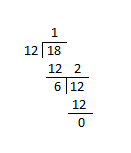# Methods of finding the highest common factor in easy steps

• Written by Millie Oscar

In this article, we’ll discuss the best methods that can be used to find out the highest common factor.

Before getting into the discussion, first, you need to know that:

## What is the Highest Common Factor HCF?

The HCF of two different numbers is the largest whole number present in both factors.

Most of the time it can recognize as the GCF “Greatest Common Factor”.

For example, the highest or greatest common factor between two random numbers 8 and 12 is 4.

## Methods to find the HCF

Mentioned below are some easiest methods to find the HCF between different numbers.1. ## Factoring Method

You have to identify all the possible factors of each number to find out the highest common factor by using the factoring method.

Factors of a specific number are the numbers that divide equally into the number with the remaining 0.

And the HCF is the highest number on every list and it also provided the list of every common factor for every number.

For example, the HCF of 18 and 45 can be calculated as:

The number 18 has the following factors:

1, 2, 3, 6, 9, and 18

And the number 25 has the following factors:

1, 3, 5, 9, 15, and 45.

The exact common factors of these two numbers are 1, 3, and 9.

Where 9 is the highest common factor of those two numbers.

1. ### Division Method

To find HCF in the division method, simply follow the mentioned below steps to find the HCF between two numbers 12 and 18:

Step 1: Take the smallest number between different numbers as the divisor and the bigger numbers as a dividend.

Step 2: Divide the divisor by the reminder to find the final results.

Step 3: Keep repeating the process until remainder becomes 0. When the remainder becomes 0, stop dividing.

For example,

The HCF of between two numbers 12 and 18 is:After dividing, the last divisor found us the HCF of the two numbers.

Also, if there is any need to find HCF between the three-number, first, find the two-number HCF and then 3rd number as well.

1. ### Finding the Prime Factors

It is one of the easiest and simple methods to find the HCF between different numbers.

Primary factors are defines as those factors of numbers which are themselves prime numbers.

You can use multiple methods to find the prime number, but one of the easiest methods is to find the tree of prime factors.

Another simple way to find the prime number is to combine the common numbers.

For example, the two random numbers are 24 and 108.

The prime factors for 24 are:

2 x 2 x 2 x 3 = 24

Whereas, the factors for 108 are:

2 x 2 x 3 x 3 x 3 = 108

You can check the common factors among these numbers are:

2 x 2 x 3 = 12

Therefore, the HCF of these two numbers is 12.

1. ### Use Online Calculators

A number of online calculators can be used to find the HCF just within a single click.

The gcd calculator provide instant results and is completely free of cost.

The calculator allow you to put two different numbers to find the HCF.

It also provide an option to select the desired method to find the greatest common factor.

1. ### Play around Method

It is also another method to find the greatest common factor.

It is considered as one of the basic methods that are used to clear the concept of finding the greatest common factor.

This method plays around with the mentioned numbers until you get the required HCF.

For example, the two different numbers are 9 and 12.

Possibilities of solutions are:

3 x 3 = 9 and 3 x 4 = 12

Here, the highest common factor among these numbers is 3.

1. ### Euclid’s Algorithm

This theorem is used to find the HCF between two different too large numbers like 123867, and 184567.

To find the HCF by using this method, all you need to do is:

• First, subtract the smaller number from the larger number from the two given numbers

• Repeat the process by subtracting the smaller number from the larger number until you get the number smaller than that of the initial number

• Then use the new initial smaller number as the new bigger number and subtract the results from the new largest number from step 2

## Last Words

HCF is the highest common factor between the two factors and is also recognized as the greatest common factor.

You can find the HCF by several manual methods like the division method or finding with the prime number method.

You also find the HCF between two numbers simply by using the online calculators.

These calculators support all the methods such as the division method, prime factoring method, and Euclid’s algorithm as well.

### LifeStyle#### Go Long: Hair Extensions 101

Hair pieces and wigs have been around since C.2700 BCE, when Egyptians used a combination of she...#### 9 Awesome Reasons to Live in Nowra

Nowra is a vibrant town, popular as Shoalhaven city’s commercial hub. It lies along the glorious...#### What are Death Doulas & What Do They Do?

Although doulas have been talked about a lot recently, they have actually been around for quite ...#### Online Activities to treat that stuck-indoors Aussie lockdown boredom

While we wait for Australian quarantines & lockdowns to reemerge in our lives somewhat like ...

# NewsServices.com

Content & Technology Connecting Global Audiences